# AP Chemistry : Oxidation-Reduction Reactions

## Example Questions

← Previous 1 3 4

### Example Question #1 : Reaction Types

What is the balanced equation when heptane is combusted?

2 C7H14 + 5 O2 → 3 H2O + 6 CO2

C7H16 + 11 O2 → 8 H2O + 7 CO2

C5H12 + 8 O2 → 6 H2O + 5 CO2

C7H16 + O2 → H2O + CO2

C3H8 + 5 O2 → 4 H2O + 3 CO2

C7H16 + 11 O2 → 8 H2O + 7 CO2

Explanation:

Heptane: C7H16. Combustion is when a molecule reacts with O2 and the products are CO2 and H2O. Balancing gives 7 CO2, 8 H2O, 1 heptane, and 11 O2

### Example Question #2 : Reaction Types

Which of the following reactions has the most exothermic heat of reaction?

The combustion of isobutane
The combustion of methane
The combustion of butane
The combustion of propane
The combustion of isopropane
Correct answer: The combustion of butane
Explanation:

The longer the hydrocarbon chain, the greater the amount of combustion products (CO2 and H20) generated. Branched molecules such as isopropane and isobutane are more difficult to combust than their straight-chain counterparts.

### Example Question #3 : Reaction Types

Which of the following conditions would describe a combustion reaction?

Exothermic with a positive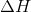Endothermic with a positiveExothermic with a negativeExergonic with a positiveEndergonic with a negativeExothermic with a negativeExplanation:

An exothermic reaction will have a negativevalue, indicating that it releases heat. Conversely, an endothermic reaction will have a positivevalue, indicating a consumption of heat.

A combustion reaction releases heat; thus it must have a negativevalue and be exothermic.

Exergonic reactions have a negative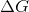value, indicating spontaneity, while endergonic reactions are non-spontaneous. While most combustion reactions will be non-spontaneous, it is impossible to draw this conclusion for certain without knowing more about the reaction. The only thing we know for certain is that heat is released, and the reaction is exothermic.

### Example Question #1 : Balancing Oxidation Reduction Reactions

The following ReDox reaction takes place in acidic solution:

Fe2+ + Cr2O72– → Fe3+ + Cr3+

What is the sum of coefficients in this redox reaction?

37

35

36

34

33

36

Explanation:

When you balance the redox reaction in acidic conditons, there are 6Fe2+, 1 Cr2O72–, 14 H+, 6 Fe3+, 2 Cr3+, and 7 H2O. Don't forget to add the 1 in front of the Cr2O72–

### Example Question #1 : Balancing Oxidation Reduction Reactions

For the following unbalanced redox reaction, how many electrons are transferred and which chemical species is being oxidized?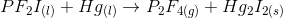One electron is transferred; P is oxidized

Two electrons are transferred; Hg is oxidized

One electron is transferred; Hg is oxidized

Two electrons are transferred; P is oxidized

Two electrons are transferred; Hg is oxidized

Explanation:

To begin, we will need to separate the given reaction into the two half-reactions by identifying changes in oxidation number. In this case, mercury (Hg) and phosphorus (P) show a change in oxidation number. Mercury begins with an oxidation number of zero, and ends with an oxidation number of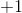. Phosphorus begins with an oxidation number of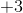and ends with an oxidation number of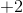. Note that the oxidation numbers for fluorine and iodine reamain constant atfor each.

Now we can begin to look at the half-reactions.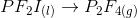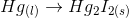Balance the atoms.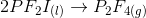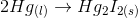Now balance the electrons. We know that each mercury atom loses one electron and each fluorine atom gains one electron.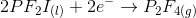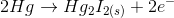We can see that two electrons are tranferred. To identify the element being oxidized, we must find the element that is losing electrons. In this case, mercury is being oxidized.

### Example Question #2 : Balancing Oxidation Reduction Reactions

How many electrons are involved in the following reaction?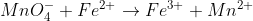1 e-

2 e-

4 e-

5 e-

3 e-

5 e-

Explanation: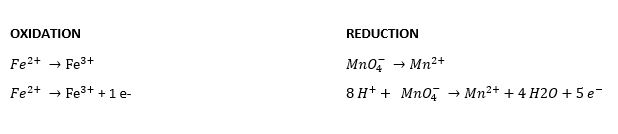The common factor between 1 e- and 5 e- is 5.  Therefore the number of electrons involved is 5 e-.

### Example Question #3 : Balancing Oxidation Reduction Reactions

How many electrons are involved in the following reaction?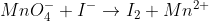4 e-

1 e-

2 e-

10 e-

5 e-

10 e-

Explanation: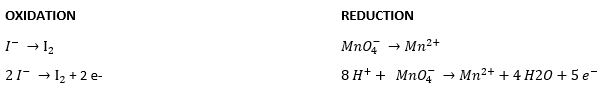The common factor between 2 e- and 5 e- is 10.  Therefore the number of electrons involved is 10 e-.

### Example Question #4 : Balancing Oxidation Reduction Reactions

What is the balanced coefficient on OH- for the following reaction: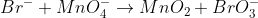(under basic conditions)

1

5

2

3

4

2

Explanation: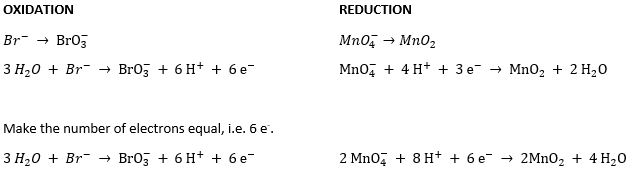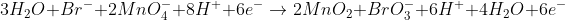Simplify: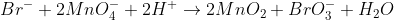Add Hydroxides to each side to counter H+.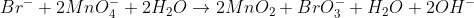Simplify: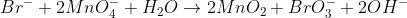### Example Question #5 : Balancing Oxidation Reduction Reactions

What is the sum of all the balanced coefficients in the following reaction: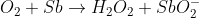(basic conditions)

10

12

8

16

14

14

Explanation: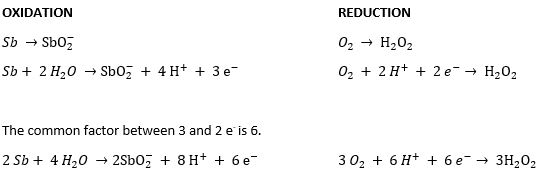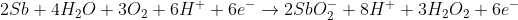Simplify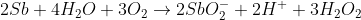Add 2 OH- to each side to cancel out the H+.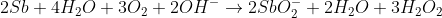Simplify: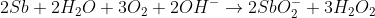### Example Question #1 : Half Reactions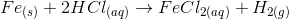For the redox reaction shown, which of the following half reactions occurs in the anode?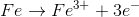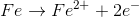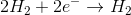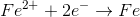Recall that oxidation always occurs at the anode (in both the electrochemical and galvanic cells).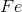loses two electrons in this case to become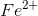. The presence ofis hinted by the ionic compound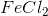.